Next: Calculating the fundamental matrix Up: Computer Vision IT412 Previous: Self-calibration and the fundamental

Subsections

# Epipolar geometry

Firstly, a word on notation. Points, as entities in their own right, will be denoted in italics. When such points are expressed in Euclidean coordinates, we will use bold notation, and when they are expressed in projective coordinates, they will be bold with a tilde. Thus a point M in three space might be imaged at m, and m might have coordinates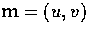or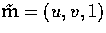.Of course, image points can also be expressed in the camera coordinate system. When we do this we will write m as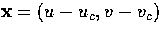or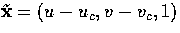.

Moreover, as with the last lecture, much of the development in this lecture is done in the setting of projective geometry, which was first introduced in Lecture 1. There is one result which we will use constantly, so it is important to have it clearly understood.

Result: A line going through two points,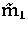and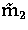is represented by the cross product.

Proof. A point on the line is given by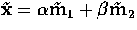, for arbitrary values of the scalars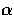and. This is equivalent to writing that the determinant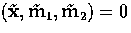.But this determinant can also be written as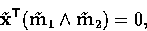so the result follows.

In the last lecture, we considered in detail the geometry of a single camera. We will now introduce a second view and study the geometric properties of the set of two views. The main new geometric property is known in computer vision as the epipolar constraint.

There are two ways of extracting three-dimensional structure from a pair of images. In the first, and classical method, known as the calibrated route, we firstly need to calibrate both cameras (or viewpoints) with respect to some world coordinate system, calculate the so-called epipolar geometry by extracting the essential matrix of the system, and from this compute the three-dimensional Euclidean structure of the imaged scene.

However it is the second, or uncalibrated route, that more likely corresponds to the way in which biological systems determine three-dimensional structure from vision. In an uncalibrated system, a quantity known as the fundamental matrix is calculated from image correspondences, and this is then used to determine the projective three-dimensional structure of the imaged scene.

In both approaches the underlying principle of binocular vision is that of triangulation. Given a single image, the three-dimensional location of any visible object point must lie on the straight line that passes through the centre of projection and the image of the object point (see figure 1). The determination of the intersection of two such lines generated from two independent images is called triangulation.Clearly, the determination of the scene position of an object point through triangulation depends upon matching the image location of the object point in one image to the location of the same object point in the other image. The process of establishing such matches between points in a pair of images is called correspondence, and will be dealt with at length in the next lecture.

At first it might seem that correspondence requires a search through the whole image, but the epipolar constraint reduces this search to a single line. To see this, we consider figure 2.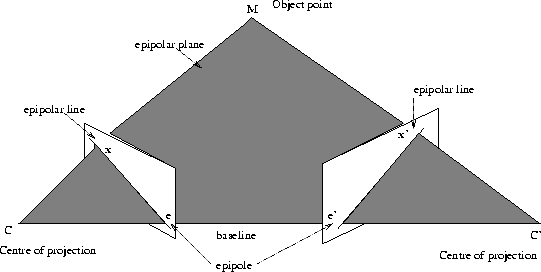The epipole is the point of intersection of the line joining the optical centres, that is the baseline, with the image plane. Thus the epipole is the image, in one camera, of the optical centre of the other camera.

The epipolar plane is the plane defined by a 3D point M and the optical centres C and C'.

The epipolar line is the straight line of intersection of the epipolar plane with the image plane. It is the image in one camera of a ray through the optical centre and image point in the other camera. All epipolar lines intersect at the epipole.

Thus, a point x in one image generates a line in the other on which its corresponding point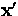must lie. We see that the search for correspondences is thus reduced from a region to a line. This is illustrated in figure 3.## The essential matrix and the fundamental matrix

To calculate depth information from a pair of images we need to compute the epipolar geometry. In the calibrated environment we capture this geometric constraint in an algebraic representation known as the essential matrix. In the uncalibrated environment, it is captured in the fundamental matrix.

With two views, the two camera coordinate systems are related by a rotation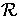and a translation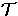(see figure 4):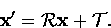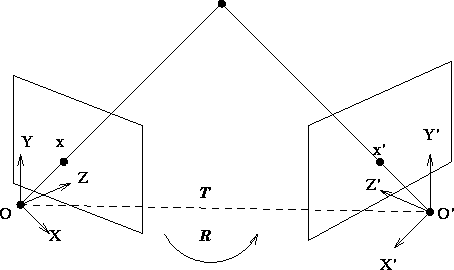Taking the vector product with, followed by the scalar product withwe obtain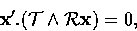(1)
which expresses the fact that the vectors Cx,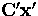and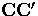are coplanar. This can also be written as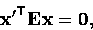(2)
where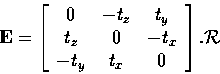is the essential matrix, and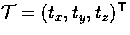.Equation (2) is the algebraic representation of epipolar geometry for known calibration, and the essential matrix relates corresponding image points expressed in the camera coordinate system.

Notice that equation (1) is homogeneous with respect to. This reflects the fact that scale is undetermined and we cannot recover the absolute scale of the scene without some extra information, such as knowing the distance in space between two points. Thusis amatrix but it only depends on five parameters. Note also that the case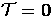is a trivial solution but one from which we are unable to calculate any information about the depth of points in space; for this reason, it is usually excluded.

In the uncalibrated case, we don't knowand; all we have are image coordinates in the image plane. Nevertheless, the correspondence between an image point m and its epipolar line lm is very simple; in fact it is linear in projective coordinates, as we can see by the following analysis.

Suppose we have two views of a point M in three dimensional space, with M imaged at m in view 1 and m' in view 2. From the last lecture we know that there are projective matricesand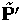such that in projective coordinates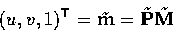andAlso, the coordinates of the two optical centres C and C' are obtained, in the world reference frame, by solving the two systems of linear equations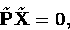and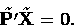Thus, since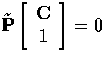, we can rewrite this as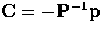, where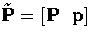.So, given a point m in the first view, its corresponding epipolar line lm can be computed from two points known to lie on it. One of these is the epipole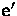and is given by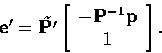Another is the point at infinity of the optical ray joining the optical centre C and the point m. The image of this point in the second image plane is given by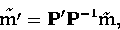because if we write the point at infinity asthen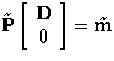and so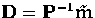.Now, the line going through two points can be represented as the cross product of those two points, so we have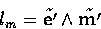and this can be written as, where F is amatrix which can be computed as follows: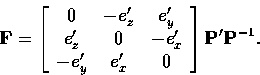Thus, there is a very clear linear relationship between a pixel and its epipolar line in projective coordinates, and this relationship is given by the fundamental matrix F. Moreover, any pixel m' on the epipolar line for m satisfies the equation(3)

Now, image points and rays in Euclidean 3-space are related by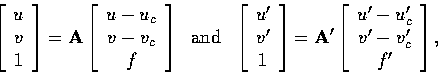so that if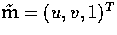and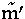is its corresponding image point, then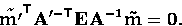Thus, when the system is calibrated, it is easy to write down the relationship between the essential and the fundamental matrices: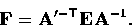The essential and the fundamental matrices have the following properties:

• the fundamental matrix encapsulates both the intrinsic and the extrinsic parameters of the camera, whilst the essential matrix encapsulates only the extrinsic parameters.
• the essential matrix E is amatrix with only 5 degrees of freedom. To estimate it using corresponding image points, the intrinsic parameters of both cameras must be known.
• F maps image points to their corresponding epipolar lines, that is,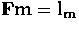, since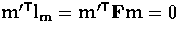. Likewise,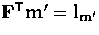since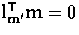.
• F maps epipoles to the origin of the corresponding image plane.
• F has 7 degrees of freedom. There are 9 matrix elements, but only their ratio is significant, which leaves 8 degrees of freedom. In addition, the constraint that detF = 0 leaves only 7.Next: Calculating the fundamental matrix Up: Computer Vision IT412 Previous: Self-calibration and the fundamental
Robyn Owens
10/29/1997